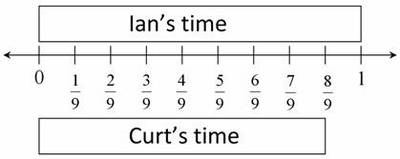# Running a Mile

Alignments to Content Standards: 5.NF.B.5

Curt and Ian both ran a mile. Curt's time was $\frac89$ Ian's time. Who ran faster? Explain and draw a picture.

## IM Commentary

There is a subtlety worth noting: we are given information about the boys' times but asked about their speeds. Since the distance they run is the same, this isn't difficult to reason through, but teachers need to be aware of this.

The two solutions reflect different competencies described in 5.NF.5. The first solution uses the idea that multiplying by a fraction less than 1 results in a smaller value. The second actually uses the meaning of multiplying by $\frac89$ to explain why multiplying by that fraction will result in a smaller value.

## Solutions

Solution: Scaling by a number less than 1

To find Curt's time, you would multiply Ian's time by $\frac89$. Since we are multiplying Ian's time by a number less than 1, Curt's time will be less than Ian's time. The picture shows Ian's time multiplied by 1 above the number line and Ian's time multiplied by $\frac89$ below the number line.Since they both ran the same distance but Curt ran it in less time, he must have been running faster.

Solution: Using the meaning of fraction multiplication

Curt's time is $\frac89 \times$ Ian's time. That means that if you divide Ian's time into 9 equal time intervals and take 8 of those intervals, you will have Curt's time. So Curt's time to run a mile is less than Ian's and he must be going faster.